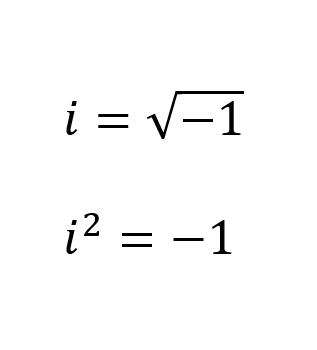Complex numbers Review

What are complex numbers?

A complex number is a number that can be expressed in the form a + bi, where a and b are real numbers, and i has a value of √-1. Because no real number satisfies √-1, i is called an imaginary number. For the complex number a + bi, a is called the real part, and b is called the imaginary part.

what are the values of i and i²?How to add or subtract complex numbers?

Add like terms For example Find the sum of (2 +3i) + (4-5i) Solution: add 2 and 4 together to obtain 6 add 3i and -5i together to obtain -2i This should result in 6-2i. You do not have to substitute √-1 into the expression.

How to multiply complex numbers.

To multiple complex numbers, multiply like you normally would, but substitute -1 for i² For example: Find the product of (2 +3i)(4-2i) using the distributive property 8 - 4i + 12i - 6i² substitute -1 for i² 8 - 4i + 12i - 6(-1) 8 - 4i + 12i + 6 The final answer is 14 - 2i

How to divide complex numbers.

multiply the the denominator and numerator of the quotient by the conjugate of the denominator, then simplify.